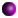Download and save the appropriate file below on your hard drive. Enter the points earned for assignments as you get them back graded and Excel will calculate your overall grade and average throughout the semester.

• Your cumulative grade is indicated in the "Current Grade" box in the last row of the table (column F).

• Your overall average is noted in the box to the right of the "To date" box on the bottom row of the table (column I): this number is an extrapolation of your actual points earned to the course's full scale of 1000 points—so for instance, the number 846.39 in this box would mean that on the scale of 1000 points you would have 846.39 points at semester's end if you maintained the exact same average in all your remaining work. Another way of reading this figure would be to move the decimal point one place to the left, which would indicate that you have an "84.639% average."

• The box in the last column on the bottom row, the "percent" column (column J), indicates what percentage of your semester grade has been computed thus far: if this box contains the figure .125, for example, this would indicate that 12.5% of your total semester grade has been calculated to this point.

• The actual number of points you have earned appears in the "Total" column (column G) on the "Total" row (the second-to-last row in the table).

Let me know if you have questions on how to use these grade calculator spreadsheets.English 1101 grade calculatorEnglish 2111 grade calculatorEnglish 2121 grade calculatorEnglish 3700 grade calculator